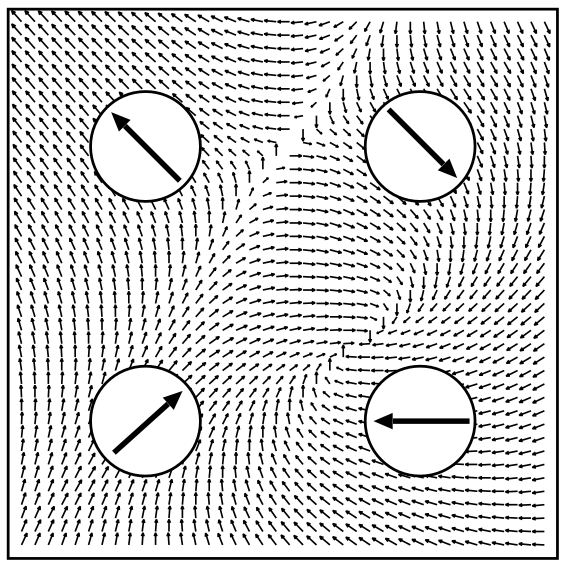## Treatment of noncollinear magnetism

In the case of noncollinear magnetism, the Kohn-Sham equation obtains the form

where ${\mathbf I}_2$ is the unit-matrix. The kinetic energy part of the Hamiltonian is diagonal in the two-dimensional spin space. Only the off-diagonal part of the hermitian $2\times2$ potential matrix

couples the two components of the Pauli spinor $\mathbf \Psi_{\nu}$. It is obtained from the density matrix, $\rho_{\alpha\beta}$, that is given by a very simple relation in terms of the solutions of the Kohn-Sham equation, $\mathbf \Psi_{\nu} = (\psi_{\nu,1},\psi_{\nu,2})$:

It is related to the density and magnetization by

where ${\mathbf I}_2$ is the unit-matrix in spin-space and $\mathbf{\sigma}$ is the vector of the Pauli matrices.

The parametrization of calculations with noncollinear magnetism requires several optional tags in the Fleur input file. To generate templates for these tags the input generator should be invoked with one of the command-line options -noco or -explicit. This will generate a nocoParams section within the calculationSetup block and further nocoParams tags in each atomGroups/atomGroup. In the Fleur input file a noncollinear calculation is activated by setting the calculationSetup/magnetism/@l_noco switch to T.

Note, that in a non-collinear calculation you can monitor the convergence of all three parts of the density matrix, the two diagonal ones (spin 1 and 2) and one of the off-diagonals (spin 3). In non-collinear calculations core-tail corrections have to be switched off: calculationSetup/coreElectrons/@ctail has to be set to F.

When performing calculations with noncollinear magnetism in Fleur the magnetization in the interstitial region is treated as a continuous vector field while in the MT spheres the magnetization is approximated to be collinear and pointing in a fixed direction. Only the magnitude of the magnetization may change within a MT sphere. This is a reasonable approach for transition metals with small spin-orbit effects. The following figure illustrates this approach.Illustration of the treatment of noncollinear magnetism in Fleur. Image taken from: Philip Kurz, Non-Collinear Magnetism at Surfaces and in Ultrathin Films (PhD Thesis, RWTH Aachen).

In the Fleur input file the direction of the magnetization in the muffin-tin spheres is specified w.r.t. the global frame for the representative atom of each atomGroup in the associated enclosed nocoParams tag. The spin-quantization axis (SQA) is provided by the angles $\alpha$ (azimuthal angle similar to $\phi$ in the context of SOC) and $\beta$ (polar angle similar to $\theta$). The example below demonstrates the nocoParams for some atom group with defined $\alpha=\pi/2.0$ and $\beta=\pi/4.0$.

<nocoParams l_relax="F" alpha="Pi/2.0" beta="Pi/4.0"
b_cons_x="0.0" b_cons_y="0.0"/>


Assume that there are two symmetry equivalent atoms in the same atomGroup of a unit cell, then setting $\alpha=0.0$ and $\beta=\pi$ describes an antiferromagnetic structure.

In detail the angles $\alpha$ and $\beta$ define the direction of the exchange $\vec{B}$ field in the atoms at the start of a self-consistency step. After the diagonalization, the resulting charge density is not necessarily collinear in the muffin-tin spheres and even the average magnetization direction can point away from the defined SQA. It can happen (e.g. due to symmetry) that the magnetization stays in the direction of the SQA, but generally this will not be the case.

To consider the full non-collinear output magnetization density (also in the MT spheres) for a plot the switch calculationSetup/nocoParams/@l_mperp has to be set to T. Afterwards one has to follow the normal plotting procedure for a density.

Note that if spin-orbit coupling is considered together with noncollinear magnetism, i.e., calculationSetup/soc/@l_soc is set to T, spin-orbit coupling is already treated in first variation. In this case calculationSetup/cutoffs/@numbands is not a relevant cutoff parameter.

Due to an ongoing generalization of the treatment of noncollinear magnetism the following calculation modes are temporarily not functional in the Fleur MaX release 4.0. They will be reactivated in future releases.

Besides just keeping the angles $\alpha$ and $\beta$ fixed there are two optional calculation modes that deal with output magnetization directions in the MT spheres that do not conicide with the input directions:

• Adjusting the SQA in the direction of the output magnetization
• Constraining the magnetization to the direction of the specified SQA

In both cases, it is necessary to know the component of the output magnetization that is perpendicular to the SQA. This is obtained by setting calculationSetup/nocoParams/@l_mperp to T.

In the first case, the relaxation of the magnetic moments, it is then sufficient to set for the respective atom groups atomGroup/nocoParams/@l_relax to T. After each iteration $\alpha$ and $\beta$ are adjusted to the output magnetization, if the mixing parameter calculationSetup/nocoParams/@mix_b is set to 1.0 completely, for smaller parameters an intermediate direction is taken (zero means no relaxation). Be aware that such a relaxation can lead to slow convergence, sometimes it helps to remove the mixing_history from time to time.

For the case of constraining the magnetization one has to set the switch calculationSetup/nocoParams/@l_constr to T. Then, a constraining field $\Delta \vec{B}^{\mu}_c$ is calculated for each atom $\mu$

which is then added to the effective $\vec{B}$ field $\vec{B}^{\mu}_{eff}$. The ratio of perpendicular magnetization, $\vec{M}^{\mu}_{\perp}$ and magnetization along the SQA, $\vec{M}^{\mu}$, is proportional to the true constraining field but the value has to be determined self consistently (with $\beta_c$ being the mixing factor mix_b). For each atom group the values of the constraining field are written as atomGroup/nocoParams/@b_cons_x and atomGroup/nocoParams/@b_cons_y in the inp.xml file for the next iteration.